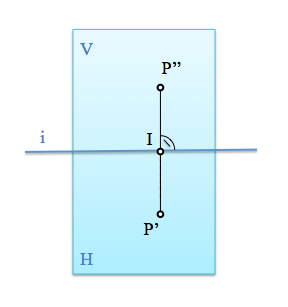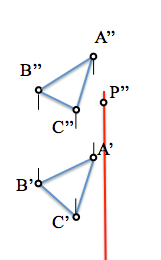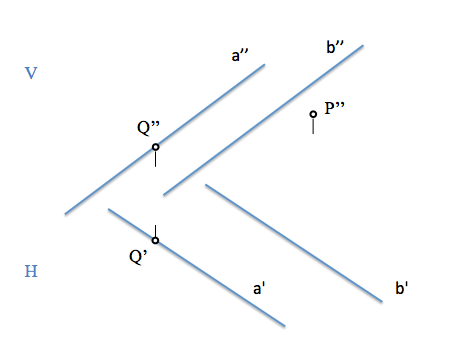# Diédrico System: Projection of points in the planeAfter seeing how projecting a plane and determine new projections We must raise the representation of elements that are on this plane, namely, We will have to determine the projections of lines and points belonging to it.

Can you get from a projection of a belonging to a flat point another projection on the plane dihedral that completes it? For example, If give us the horizontal projection and vertical of a plane and a point in the latter as determinaríamos the projection on the horizontal plane?This is the most basic of the membership problems.

By studying the Fundamentals del System dihedral We have seen the relationship between prospective between projections diedricas, in such a way that we can determine a prime locus in which must be the projection that we are looking for the point P.Therefore the sought after screening will be on one reference line It relates the Horizontal and Vertical planes, stop by the projection of the point P”.To solve the problem, we must find a new locus in which is situated the sought after screening, solving the problem by means of the intersection of both loci.

By a point in a plane infinite straight pass. Straight such projections will pass through the projection of the point that contains them.

We will build on a straight either passing through the point P and which belong to the plane defined by the points A, B and C.The line “r” that has drawn through one of the points of the plane (B) and for its P, in the vertical projection, If you belong to the plane it will cut their lines; point “1” the straight is the intersection with the straight A-C.

To locate this line in the horizontal projection must find the projection that is missing the point “1“, looking for your reference line knowing that you must be on the straight line projections A-C.The sought after projection of the point P you will find on the projection of the line.

We see that we have simultaneously solved the determination of a point, P, and a straight, r, they are located in a plane and that they are determined by a unique projection and the membership condition. We will see that there are certain lines that have a spatial position that makes them especially interesting to work with drawings. We will call these lines “notable lines of plane

I leave a similar to the previous exercise to reinforce concepts:

Can you determine the first projection of the point P that belongs to the plane defined by the straight lines “to” and “b”?Sistemas_de_representacion